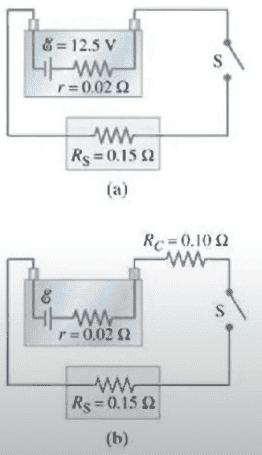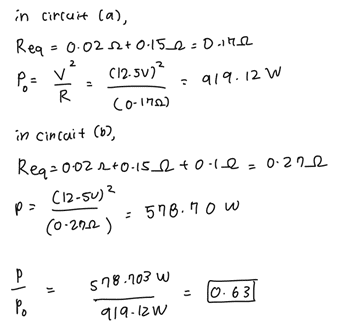# DC circuit finding Req

• Sunwoo Bae
In summary, the conversation discusses the concept of series circuits and how they are defined by the flow of current through multiple components in a single path. It also addresses a mistake in the question regarding the calculation of power delivered to a circuit with corrosion. The conversation also mentions the possibility of circuits that cannot be classified as series or parallel.f

#### Sunwoo Bae

Homework Statement
The performance of the starter circuit in an automobile can be significantly degraded by a small amount of corrosion on a battery terminal. Figure 26-38a depicts a properly functioning circuit with a battery (12.5-V emf, 0,02 ohms internal resistance) attached via corrosion-free cables to a starter motor of resistance Rs = 0.15 ohms. Suppose that later, corrosion between a battery terminal and a starter cable introduces an extra series resistance of just Rc = 0.10 ohms into the circuit as suggested in figure. Let P_0 be the power delivered to the starter in the circuit free of corrosion, and let P be the power delivered to the circuit with corrosion. Determine P/P0.
Relevant Equations
Resistors in parallel/ Resistors in seriesThe answer sheet assumes that the resistors in both circuits are in series and that we need to use the equation Req = R1+R2+R3.. to find the Req in both cases. How come the resistors are in series, when there are multiple resistors in a single row?

Also, why does the following work yield the wrong answer? The answer should be 0.4, but I keep getting 0.63..How come the resistors are in series,
Because the current flowing through all of them is the same.

You need to calculate the power delivered to "the starter". Is it the same as what you have calculated?

•Steve4Physics
I'd like to add to what @cnh1995 has said.

Components are in series when connected one after the other, in a simple 'chain'. All the current leaving a component enters the next component in the chain. So the current is never split.

There is a mistake in the question.
... and let P be the power delivered to the circuit with corrosion.
It should say:
"... and let P be the power delivered to the starter in the circuit with corrosion."

•phinds
How come the resistors are in series, when there are multiple resistors in a single row?
Uh ... 'cause that's the DEFINITION of series?

Hi Sunwoo Bae,

If the current flows through your components in a single loop or a single path then it is a series circuit.
If the current flows through your components in two or more loops or paths then it is a parallel circuit.

Think of it as if you are walking through a forest, if :
1- There's only one path = series (for all components along that path)
2- There are multiple paths = parallel (for the components on that are on different paths)

GJ

Hi Sunwoo Bae,

If the current flows through your components in a single loop or a single path then it is a series circuit.
If the current flows through your components in two or more loops or paths then it is a parallel circuit.

Think of it as if you are walking through a forest, if :
1- There's only one path = series (for all components along that path)
2- There are multiple paths = parallel (for the components on that are on different paths)
Hi @Guy Joel R. Welcome to PF!

In case you hadn't noticed, this is an old thread, dating back to February!

It may also be worth noting that not all circuits can be claasified as series or parallel or some combination of these - the classic example being a bridge circuit.